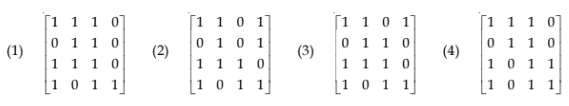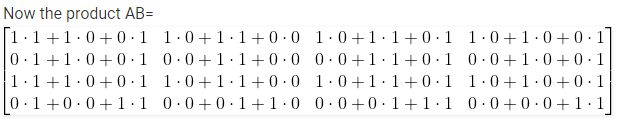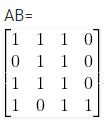# UGC NET COMPUTER SCIENCE SOLVED PAPERS 2017-19 - UGC NET Computer Science November 2017 Paper 2

>>>>>>>>UGC NET Computer Science November 2017 Paper 2

• A

9 O’clock• B

8 O’clock• C

5 O’clock• D

4 O’clock• Option : A
• Explanation :
We know that there are 24 hours in a day, so we will take mod for 101 hours ie (101 mod 24) = 5. So residue is 5. On adding these remaining 5 hours to the present time will give 9 O'CLOCK time.

• A

(635)4• B

(32312)4• C

(21323)4• D

(1301)4• Option : D
• Explanation :
We have m=(313)4 and n=(322)4
convert m and n into decimal: m = 3*42 + 1*41 + 3*40 m = 48 + 4 + 3 m = 55.
Now n=3*42 + 2*41 +2*40   n = 48 + 8 +2   n = 58. m + n = 55 + 58 m + n = 113
Now we have to convert 113 in to base 4: ie step 1-113 % 4 = 1  113 / 4 = 28 step 2- 28 % 4 = 0   28 / 4 = 7 step 3- 7 % 4 = 3   7 / 4 = 1 step 4- 1 % 4 = 1   1 / 4 --> will not divide it in quant.
So we have to stop here. The answer will be Residue from step 4 to step 1 inorder ie 1301 Ans-(1301)4• A

(1)• B

(2)• C

(3)• D

(4)• Option : A
• Explanation :

Here we have to follow two basics: 1- Matrics multiplication rule- For multiplication of two matrics, column in first matrics must be equal to rows in second matrics. 2- We have to find boolean product of two matrics so, we must know boolean product ie

 A B F 0 0 0 0 1 0 1 0 0 1 1 1

Now we haveSo, option (A) is correct.

• A

720• B

120• C

60• D

360• Option : C
• Explanation :
In BANANA we have six letters in total but here we have some duplicate letters too so we have to deal with it and have to remove those duplicate case. B - 1 A - 3 N - 2 So total no of words possible is factorial(6) ie 6! but we must remove duplicate words: ie- (6!/(2!*3!)) which gives 60 So 60 distinguishable permutation of the letters in BANANA. So, option (A) is correct.

Use Kruskal’s algorithm to find a minimal spanning tree for the graph. The List of the edges of the tree in the order in which they are choosen is?
(1) AD, AE, AG, GC, GB, BF
(2) GC, GB, BF, GA, AD, AE
(3) GC, AD, GB, GA, BF, AE
(4) AD, AG, GC, AE, GB, BF

• A

(1)• B

(1),(2)• C

(1), (2), (3)• D

(1), (2), (3), (4)• Option : D
• Explanation :
Using Kruskal's algorithm for minimal spanning tree: First select the minimum weight edge ie 2 we have two options GC and AD Now select Next edge of minimum weight: for GC we have to select GB or AD and so on select minimum weight keep in mind no vertex left to visit. Here all option are matching with minimum spanning tree. So, option (D) is correct.